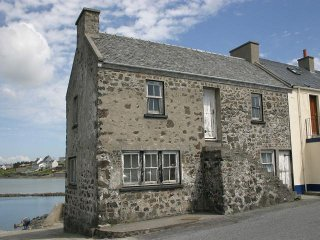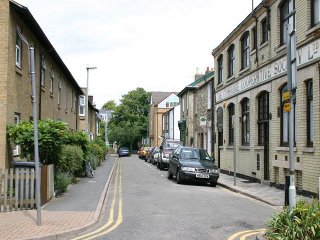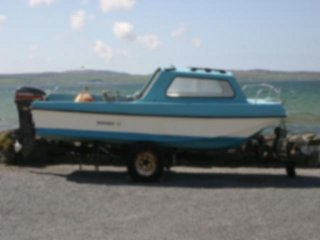## Image Blur Detection via Hough Transform — IV

In my previous three articles (1,2,3) I discussed how to use Canny edge detection and Hough transform to identify blur images. Here I will show some results from the algorithm discussed before.

### Results

When presented with images that are clear, the algorithm correctly identified most of them (see images below):Building (Microsoft Research Digital Image)Street (Microsoft Research Digital Image)

The following images illustrate how the original image (top right) is divided into sub regions. Canny detection is performed on each of the sub images.

When performing Hough Transform, I chose to detect up to ten lines in each image, with the following stepping parameter (the detection results are very sensitive to these parameters, the following parameters were chosen based on experiment results):
$\rho=1, \theta=0.01$

Out of all the detected lines, a few sections are selected based on line continuity and the calculated average gradients around the detected lines. The following images shows the chosen Hough line segments based on the algorithm.

The table below shows the gradient index calculated within each area (the average of the gradients along all the chosen line segments within a sub-image. The result is scaled by 1000, the scale factor is chosen such that for clear images the resulted index is greater than 1 and for blur images the resulted index is less than 1).

 1 1.709 2 2.383 3 1.012 4 2.842 5 3.389 6 2.419 7 2.933 8 2.168 9 2.534

And the sub images are indexed as follows:

 1 4 7 2 5 8 3 6 9

The algorithm can also detect images with deliberate blur regions (e.g. Bokeh). The results are illustrated below:

 1 0 2 1.75 3 1.973 4 1.595 5 2.815 6 3.188 7 0 8 1.204 9 1.308

Note that the 0’s in the detection results indicate that within those regions, no lines could be reliably detected and thus those regions are considered blurred.
Generally speaking, when an image contains both blurred and clear regions, some of the indexes will be zero and others will be greater than one.

The following images are detected as blurred, with the detected indexes far less than one.Boat (Microsoft Research Digital Image)

### Limitations

when image contrast is low, or when objects borders are not clearly defined, the algorithm may have difficulty in distinguishing whether an image is blurred. Take the following two cloud images for instance, the image on the left was correctly classified as a clear image due to the relatively high contrast around the center. But the image to the right was classified as blurred due to its lack of contrast.

### Update (7/12/2010)

A few readers requested the code for calculating gradients. The method I used was quite simple, please see the LineUtils code below:

/*
* File:   lineutils.cpp
* Author: kwong
*
* Created on June 5, 2009, 8:47 PM
*/

#include "LineUtils.h"

#include <math.h>
#include <stdlib.h>
#include <iostream>
#include <ios>

using namespace std;

namespace KDW {
const float PI = 3.14159265358979;
const float Epsilon = 1e-5;
const float MaxGradient = 1e6;

LineUtils::LineUtils() {
}

LineUtils::LineUtils(const LineUtils& orig) {
}

LineUtils::~LineUtils() {
}

/**
* Get the equation parameters for the line that passes through (x,y) and is perpendicular
* to the line specified by parameters (p0, theta0) in normal form.
*
* @param p0 : distance to line from origin.
* @param theta0 : the slope of p0.
* @param x : x coordinate of the point where the perpendicular line passes through
* @param y : y coordinate of the point where the perpendicular line passes through
* @param &p : the perpendicular line's distance from origin.
* @param &theta : the slope of p.
**/
void LineUtils::GetPerpendicularLineParameters(float p0, float theta0, float x, float y, float &p, float &theta) {
float x0 = p0 * cos(theta0);
float y0 = p0 * sin(theta0);

p = sqrt((x0 - x)*(x0 - x) + (y0 - y)*(y0 - y));

float a1 = theta0 - PI / 2.0;
float a2 = theta0 + PI / 2.0;

//d=|x0 * cos a + y0 * sin a - p|
float d1 = abs(x * cos(a1) + y * sin(a1) - p);
float d2 = abs(x * cos(a2) + y * sin(a2) - p);

if (d1 < d2)
theta = a1;
else
theta = a2;
}

/**
* Get a line segement's coordinates. The segement is centered at x0, y0.
*
* @param p : distance to line from origin.
* @param theta : the slope of p.
* @param x0 : x coordinate of the segement center.
* @param y0 : y coordinate of the segement center.
* @param numOfPoints : the number of coordinate points to collect at either side
*                      of (x0, y0)
* @param points[] : returns the x,y coordinates of the points on the line segement in IppiPoint.
**/
void LineUtils::GetLineIntervalPoints(float p, float theta, float x0, float y0, int numOfPoints, IppiPoint points[]) {
if (abs(sin(theta)) < Epsilon) {
for (int xp = x0 - numOfPoints; xp <= x0 + numOfPoints; xp++) {
points[(int) (xp + numOfPoints - x0)].x = xp;
points[(int) (xp + numOfPoints - x0)].y = y0;
}
} else if (abs(cos(theta)) < Epsilon) {
for (int yp = y0 - numOfPoints; yp <= y0 + numOfPoints; yp++) {
points[(int) (yp + numOfPoints - y0)].y = yp;
points[(int) (yp + numOfPoints - y0)].x = x0;
}
} else if ((abs(theta) > 0 && abs(theta) <= 0.25 * PI) ||
(abs(theta) > 0.75 * PI && abs(theta) <= PI) ||
(theta > PI && theta <= 1.25 * PI) ||
(theta > 1.75 * PI and theta <= 2.0 * PI)) {
for (int xp = x0 - numOfPoints; xp <= x0 + numOfPoints; xp++) {
points[(int) (xp + numOfPoints - x0)].x = xp;
points[(int) (xp + numOfPoints - x0)].y = (int) ((p - points[(int) (xp + numOfPoints - x0)].x * cos(theta)) / sin(theta));
}
} else {
for (int yp = y0 - numOfPoints; yp <= y0 + numOfPoints; yp++) {
points[(int) (yp + numOfPoints - y0)].y = yp;
points[(int) (yp + numOfPoints - y0)].x = (p - points[(int) (yp + numOfPoints - y0)].y * sin(theta)) / cos(theta);
}
}
}

/**
* Get a line segement's coordinates. The segement is centered at x0, y0.
*
* @param points[] : the x,y coordinate of the input points (ordered).
* @param threashold : the threashold of disconnected points before a line is considered no
*              longer connected.
* @param lpoints[] : the x,y coordinates of the points in the detected longest connected line.
* @param &maxLen : the maximum length of the output array, addtional line points
*                  will be discarded if exceed this limit.
**/
void LineUtils::GetLongestConnectedLinePoints(IppiPoint points[], int len, int threshold, IppiPoint lpoints[], int &maxLen) {
int maxIndexEnd = 0, curRunLength = 0, maxRunLength = 0;

lpoints[curRunLength] = points;

for (int i = 1; i < len; i++) {
//if ((abs(points[i].x - points[i - 1].x) > threshold) || (abs(points[i].y - points[i - 1].y) > threshold)) {
if ((abs(points[i].x - lpoints[curRunLength].x) > threshold) || (abs(points[i].y - lpoints[curRunLength].y) > threshold)) {
//if (curRunLength > maxRunLength) {
if (curRunLength > maxRunLength) {
maxRunLength = curRunLength;
maxIndexEnd = i;
}
curRunLength = 0;
lpoints[curRunLength] = points[i];

//}
} else {
if (curRunLength >= maxLen) {
maxRunLength = maxLen;
break;
}

curRunLength++;
lpoints[curRunLength] = points[i];
}
}

for (int i = maxIndexEnd - maxRunLength - 1; i < maxIndexEnd; i++) {
if (i - (maxIndexEnd - maxRunLength) - 1 < maxRunLength) {
lpoints[i - (maxIndexEnd - maxRunLength - 1)] = points[i];
}
}

maxLen = maxRunLength;
}

bool LineUtils::GetMinMaxIndicies(float pixels[], int len, int &minIndex, int &maxIndex) {
bool isEdge = false;
bool isPositive = true;

int numCrossings = 0;

minIndex = 0;
maxIndex = 0;

float avg = 0;

for (int i = 0; i < len; i++) {
avg += pixels[i];
}

avg = avg / (float) len;

if (pixels > avg)
isPositive = true;
else
isPositive = false;

for (int i = 0; i < len; i++) {
if (pixels[i] < pixels[minIndex]) {
minIndex = i;
} else if (pixels[i] > pixels[maxIndex]) {
maxIndex = i;
}

if (isPositive && pixels[i] < avg) {
isPositive = false;
numCrossings++;
} else if (!isPositive && pixels[i] > avg) {
isPositive = true;
numCrossings++;
}
}

if (numCrossings < 3)
return true;
else
return false;
}

float LineUtils::CalculateGradient(IppiPoint points[], float pixels[], int len, int minIndex, int maxIndex) {
float lAvg = 0;
float hAvg = 0;

if (minIndex > 0 && minIndex < len - 1) {
lAvg = (pixels[minIndex] + pixels[minIndex - 1] + pixels[minIndex + 1]) / 3.0;
} else if (minIndex > 1) {
lAvg = (pixels[minIndex] + pixels[minIndex - 1] + pixels[minIndex - 2]) / 3.0;
} else if (minIndex < len - 2) {
lAvg = (pixels[minIndex] + pixels[minIndex + 1] + pixels[minIndex + 2]) / 3.0;
} else {
lAvg = pixels[minIndex];
}

if (maxIndex > 0 && maxIndex < len - 1) {
hAvg = (pixels[maxIndex] + pixels[maxIndex - 1] + pixels[maxIndex + 1]) / 3.0;
} else if (maxIndex > 1) {
hAvg = (pixels[maxIndex] + pixels[maxIndex - 1] + pixels[maxIndex - 2]) / 3.0;
} else if (maxIndex < len - 2) {
hAvg = (pixels[maxIndex] + pixels[maxIndex + 1] + pixels[maxIndex + 2]) / 3.0;
} else {
hAvg = pixels[maxIndex];
}

float h = hAvg - lAvg;
float t = abs(h / (float) (points[maxIndex].x - points[minIndex].x));

if (t > 0)
return t > MaxGradient ? MaxGradient : t;
else
}

float LineUtils::GetAverage(float gradients[], int count) {
int zerosCount = 0;
int minValIndex = 0, maxValIndex = 0;

for (int i = 0; i < count; i++) {
if (gradients[i] == 0)
zerosCount++;

maxValIndex = i;
minValIndex = i;
}

zerosCount += 2;

if (zerosCount < 6) {
for (int i = 0; i < count; i++) {
maxValIndex = i;
minValIndex = i;
}
}

zerosCount += 2;

if (zerosCount < 6) {
for (int i = 0; i < count; i++) {
maxValIndex = i;
minValIndex = i;
}
}

float r = 0;
zerosCount = 0;
for (int i = 0; i < count; i++) {
if (gradients[i] > 0) {
zerosCount++;
}
}

if (zerosCount == 0)
return 0;
else
return r / (float) zerosCount;
}
}


1.Antonin JACQ says:

Hi.

I am currently a student at the university of La Rochelle, France, in an image processing & mathematics master.
Our teachers asked us to build several image default detector: noise(salt&pepper, ccd), block artifact, color dominance, motion-blur and out-of-focus blur.

I am working since yesterday on your articles for blur detection via Hough Transform.

Working on Matlab, i succesfully reproduce almost everything, except for the final part of the algorythm: the calcul of the gradient on the line.
I didn’t understand what you are doing:
– in which image is this line? In the Hough transform? In the orignal image?
– Similarly, what is the use of the perpendicular line whom you speak of for the gradient calculation?

My principal problem lies with the definition of the gradient D of an image :
In classics way, D=[Ix,Iy] with Ix and Iy being a vector field (Ix(x,y)=I(x,y)-I(x-1,y), the same for Iy). Here i do not know what is it.

If you’re willing to enlight me, i would be thankful.

Antonin.

•kwong says:

The gradient calculation involves fitting a line through the detected edge points. After the linear equation is obtained, calculating the perpendicular line is simple. Similarly, the gradient can be calculated by modeling the intensity as a linear function (the simplest method) and the gradient would be the tangent of the linear function. Hope this helps.

2.andrey says:

Hi from Russia!
I am author of ippiHoughLine_8u32f_C1R function.
Thank you for using Intel IPP library.
I hope is is usefull for you!

•kwong says:

Thanks Andrey!

3.samcal says:

I’m trying to implement this using OpenCV, which also does Canny edge detection and Hough line detection.

I have my Hough-paramaterised lines but I’m still unclear about how you calculate the gradient in the 10-pixel area around the line segment (in the window which is given by d.)

Could you elaborate on how you implemented the gradient calculation element of the system?

Many thanks for your help.

•kwong says:

Hi samcal,

I just updated the article above to include the LineUtil class which contains the gradient calculation. Hope it helps.

•david stone says:

Did you ever get this working with OpenCV? I’m using OpenCV for face detection and would like to be able to detect how blurry the face is as well but havn’t found an implementation for it yet.

4.alexis says:

I am trying to detect blurred images. before i thought it was impossible yet i stumble with your work. is there a way i can transpose everything to C# i am using aforge.net and emgucv a wrapper class for opencv. really need your help with this one. thanks.

5.Nyson says:

Thanks for this enlightening article, it is really quite interesting, previously I would not have considered edge detection for determining blur, however it seems so obvious now.

You also showed some situations in which this approach fails, are there any other methods that might get around these shortcomings that you are aware of?

Thanks for sharing.

6.sur says:

Hello Kwong,
I’m really thankful for your article on Blur detection. As i didn’t understand the code completely i would like to have a look at LineUtils.h header file. Can you post it or mail it to animatsurendra(at)gmail(dot)com

Thany you..

•kwong says:

Sure thing. Here’s the header file:

/*
* File: lineutils.h
* Author: kwong
*
* Created on June 5, 2009, 8:47 PM
*/

#ifndef _LINEUTILS_H
#define _LINEUTILS_H

#include

using namespace std;

namespace KDW {
class LineUtils {
public:

LineUtils();
LineUtils(const LineUtils& orig);
virtual ~LineUtils();

void GetLineIntervalPoints(float p, float theta, float x0, float y0, int numOfPoints, IppiPoint points[]);
void GetPerpendicularLineParameters(float p0, float theta0, float x, float y, float &p, float &theta);
void GetLongestConnectedLinePoints(IppiPoint points[], int len, int threshold, IppiPoint lpoints[], int &maxLen);
bool GetMinMaxIndicies(float pixels[], int len, int &minIndex, int &maxIndex);
float CalculateGradient(IppiPoint points[], float pixels[], int len, int minIndex, int maxIndex);
float GetAverage(float gradients[], int count);
private:
};
}
#endif /* _LINEUTILS_H */

7.theodore says:

Hi Kerry,

i am working in project right now that one part of it, is related with blur detection and i have seen your article about detecting blur in images by using hough transform

http://www.kerrywong.com/2009/07/03/image-blur-detection-via-hough-transform-iv/

i think that your implementation is quite interesting and i would like to try it. My question are if it possible to have an archive of the code and if you will be able to give me a feedback to some questions that i have at the moments. Since right now i am trying to implement your thoughts by using the OpenCV library.

Thanks in advance. Looking forward to hearing from you.

8.Gergely Mezei says:

Hi!

Is it possible to obtain the whole source code? I would gladly port it to Visual Studio environment in return (thus it can be published to several platforms later). I would like to create a pre-filtering mechanism to organize my digital images, that’s why I am interested in your project.

9.Anurag Prabhakar says: